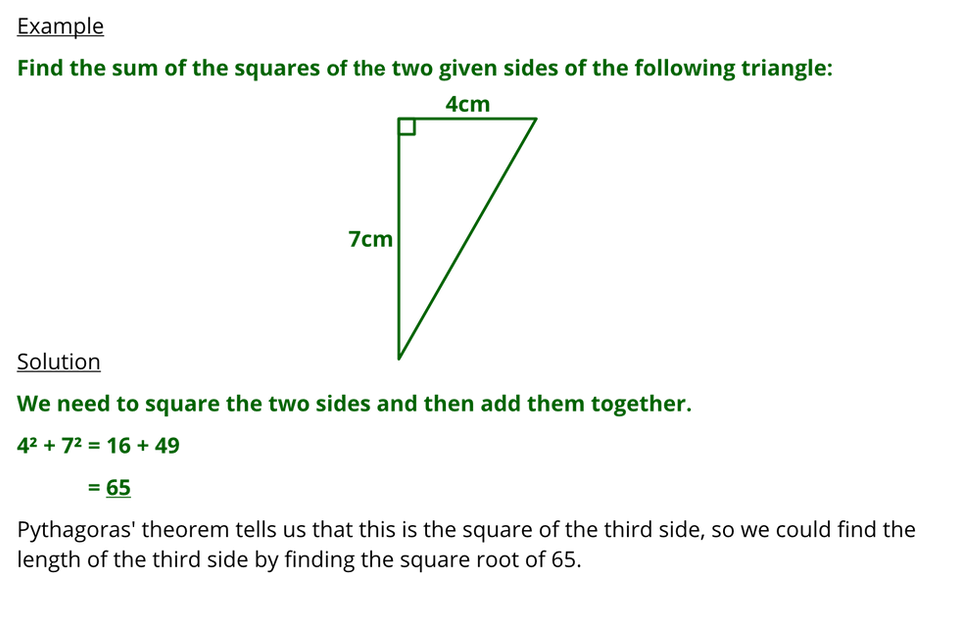Sample lesson

Pythagoras' Theorem 1

Progress:

5

10

4

10

You should use a calculator for this lessonThe notes are hidden when you only need 1 or 2 correct answers to complete the lesson. Also you cannot see the worked solutions  or  have  the  easier  questions.

Find the length of the side marked 𝑥. Give your answer correct to 3 significant figures.

Find the length of the side marked 𝑥

Find the sum of the squares of the two given sides:

5cm

10cm

𝑥𝑥5cm

10cm

5cm

𝑥10cm

10cm

𝑥5cm

cm

Correct

This is not correct.
𝑥² = 16² + 7²
= 256 + 49
= 305
𝑥 = √305
= 17.5cm  (to 3 s.f.)

𝑥² = 9² + 5²
= 81 + 25
= 106
𝑥 = √106
= 10.3cm  (to 3 s.f.)

𝑥² = 23² + 11²
= 529 + 121
= 650
𝑥 = √650
= 25.5cm  (to 3 s.f.)

𝑥² = 15² + 7²
= 225 + 49
= 274
𝑥 = √274
= 16.6cm  (to 3 s.f.)

𝑥² = 2² + 1²
= 4 + 1
= 5
𝑥 = √5
= 2.24cm  (to 3 s.f.)

This is not correct.
𝑥² = 10² + 6²
= 100 + 36
= 136
𝑥 = √136
= 11.7cm  (to 3 s.f.)

We are finding the longest side so we need

to square the other two sides and add them.

This is not correct.
𝑥² = 16² + 8²
= 256 + 64
= 320
𝑥 = √320
= 17.9cm  (to 3 s.f.)

You didn't square the 16.

This is not correct.
𝑥² = 21² + 9²
= 441 + 81
= 522
𝑥 = √522
= 22.8cm  (to 3 s.f.)

You didn't square the 9.

This is not correct.
𝑥² = 2² + 1²
= 4 + 1
= 5
𝑥 = √5
= 2.24cm  (to 3 s.f.)

You didn't square root the 5.

This is not correct.
9² + 4² = 81 + 16
= 97

This is not correct.
17² + 8² = 289 + 64
= 353

This is not correct.
21² + 11² = 441 + 121
= 562

This is not correct.
12² + 6² = 144 + 36
= 180

We are finding the sum of the squares

so the squares need to be added. You